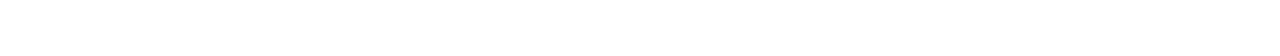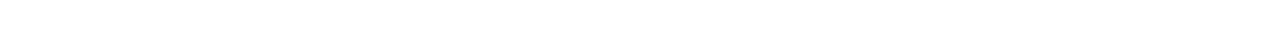# 16.1.6 Average Kinetic Energy of a Molecule

### Average Kinetic Energy of a Molecule

• An important property of molecules in a gas is their average kinetic energy
• This can be deduced from the ideal gas equations relating pressure, volume, temperature and speed
• Recall the ideal gas equation:

pV = NkT

• Also recall the equation linking pressure and mean square speed of the molecules:
• Multiplying both sides of the equation by ½ obtains the average translational kinetic energy of the molecules of an ideal gas:• Where:
• EK = kinetic energy of a molecule (J)
• m = mass of one molecule (kg)
• <c2> = mean square speed of a molecule (m2 s-2)
• k = Boltzmann constant
• T = temperature of the gas (K)
• Note: this is the average kinetic energy for only one molecule of the gas
• A key feature of this equation is that the mean kinetic energy of an ideal gas molecule is proportional to its thermodynamic temperature

EK ∝ T

• Translational kinetic energy is defined as:

The energy a molecule has as it moves from one point to another

• A monatomic (one atom) molecule only has translational energy, whilst a diatomic (two-atom) molecule has both translational and rotational energy

Step 2:            Find the relation between cr.m.s and temperature T

Since m and k are constant, <c2> is directly proportional to T

<c2> ∝ T

Therefore, the relation between cr.m.s and T is:Step 3:            Write the equation in fullwhere a is the constant of proportionality

Step 4:            Calculate the constant of proportionality from values given by rearranging for a:

T = 45 oC + 273.15 = 318.15 K

#### Exam Tip

Keep in mind this particular equation for kinetic energy is only for one molecule in the gas. If you want to find the kinetic energy for all the molecules, remember to multiply by N, the total number of molecules.

You can remember the equation through the rhyme ‘Average K.E is three-halves kT’.### Author: Katie

Katie has always been passionate about the sciences, and completed a degree in Astrophysics at Sheffield University. She decided that she wanted to inspire other young people, so moved to Bristol to complete a PGCE in Secondary Science. She particularly loves creating fun and absorbing materials to help students achieve their exam potential.
CloseClose